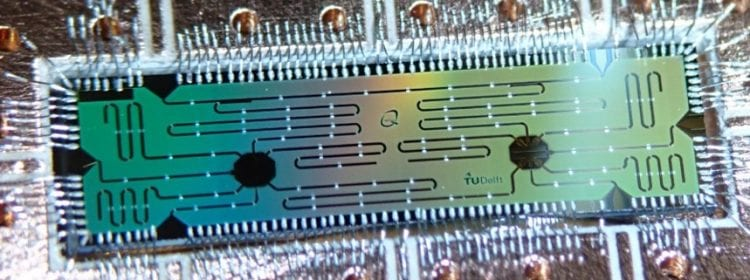QuTechScalable quantum circuit and control for a superconducting surface code

Quantum computers offer and enormous speed advantage over classical computers for several problems of practical interest. This speed advantage can even scale exponentially with the number of qubits, the quantum-mechanical equivalent of a bit, offering computing power that will potentially outperform any classical computer. The basic building blocks to perform quantum algorithms on a quantum computer, such as single-qubit gates, two-qubit gates and qubit measurements, have been demonstrated. However, scalability issues such as performing two-qubit gates on many qubits simultaneously must be overcome to keep increasing the number of qubits on-chip.

Scalable scheme
Researchers at QuTech developed a scalable scheme for executing the error correction cycle of a monolithic surface code composed of fast-flux-tuneable transmon qubits with nearest-neighbor couplings. An eight-qubit unit cell forms the basis for repeating both the quantum hardware and coherent control, enabling spatial multiplexing with a fixed set of control components. The scheme performs the surface code quantum error correction cycle and operations on logical qubits encoded in physical qubits.

The work presented combines four key elements, leading to a scalable solution: 1) the definition of a unit cell, consisting of 8 qubits, from which a surface code of arbitrary size can be assembled by repetition, 2) a specific order to execute two-qubit gates (CZ gates) and parity measurements in a pipelined fashion, which maximally de-couples the two types of parity checks in the surface code, 3) specific single-qubit and two-qubit operation frequencies and 4) construction of two-qubit operation control signals from three primitive control pulses, multiplexed to the unit cells. This solution offers an integrated basic building block for fault-tolerant quantum computation.

Reference: Scalable quantum circuit and control for a superconducting surface code, R. Versluis, S. Poletto, N. Khammassi, N. Haider, D. J. Michalak, A. Bruno, K. Bertels, L. DiCarlo, arXiv:1612.08208 [quant-ph]